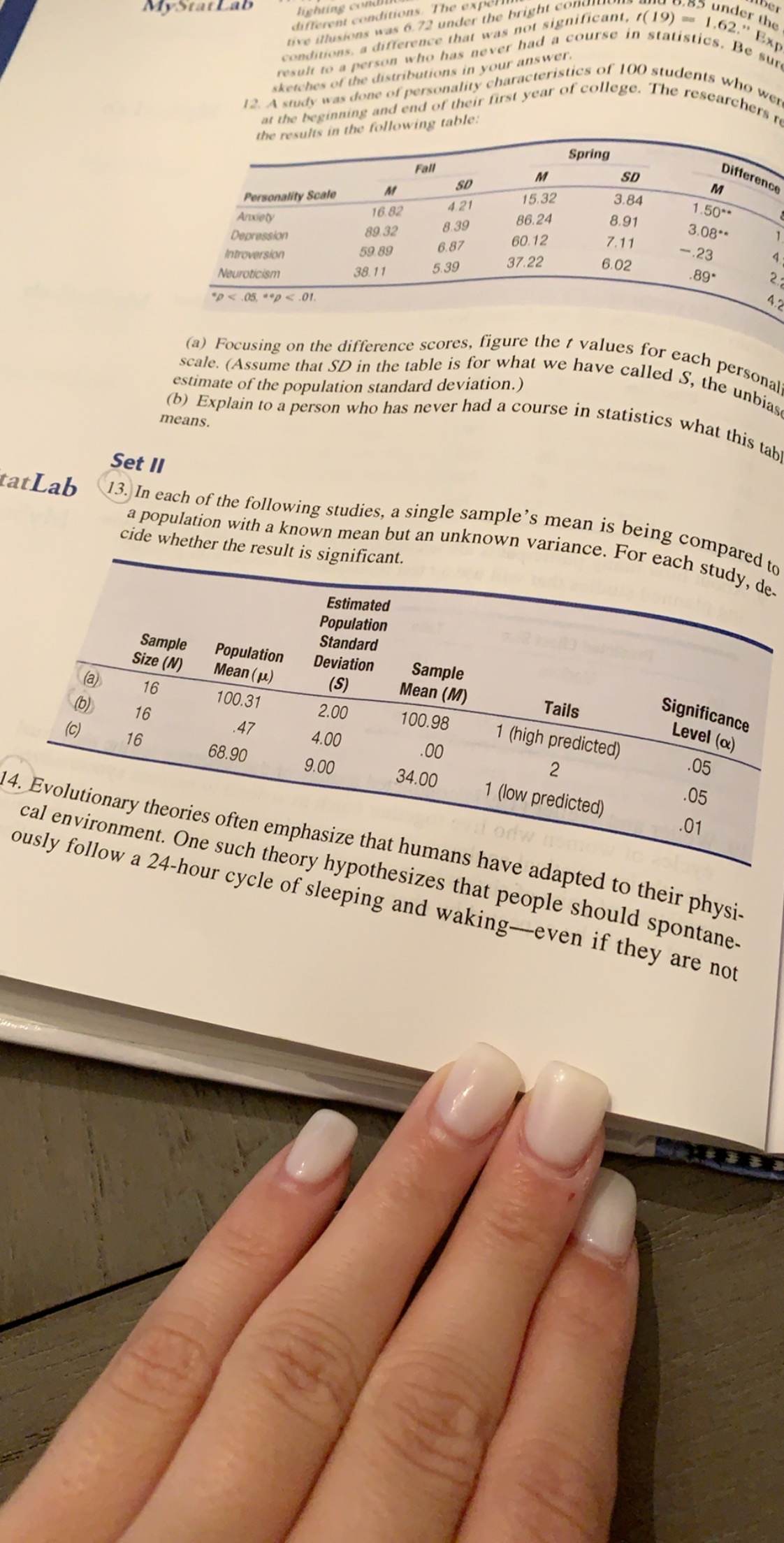# berunder theighting con.different conditions The expetive illusions was 6.72 under the bright coMyStatLabconditions, a difference that was not significant, r(19)-1.62." Expresult to a person who has never had a course in statistics. Be surresearchers reThe'collegeat the beginning and end of their first year ofthe results in the following table12 A study was done of personality characteristics of 100 students who weresketches of the distributions in your answerDifferenceSpringSDMFall3.84SD1.50*15.32Personality ScaleAnxietyDepression4.218.913.08186.2416.828.397.11-2360.1289 326.876.022289*37.2259 895.394.2Introversion38.11NeuroticismDA 05,p

Question

In each of the following studies, a single samples mean is being compared to a population with a known mean but an unknown variance. For each study, decide whether thee result is significnt.  (only a and b)help_outlineImage Transcriptioncloseber under the ighting con. different conditions The expe tive illusions was 6.72 under the bright co MyStatLab conditions, a difference that was not significant, r(19)-1.62." Exp result to a person who has never had a course in statistics. Be sur researchers re The 'college at the beginning and end of their first year of the results in the following table 12 A study was done of personality characteristics of 100 students who were sketches of the distributions in your answer Difference Spring SD M Fall 3.84 SD 1.50* 15.32 Personality Scale Anxiety Depression 4.21 8.91 3.08 1 86.24 16.82 8.39 7.11 -23 60.12 89 32 6.87 6.02 22 89* 37.22 59 89 5.39 4.2 Introversion 38.11 Neuroticism DA 05,p<.01 (a) Focusing on the difference scores, figure the t values for each personal scale. (Assume that SD in the table is for what we have called S, the unbiase (b) Explain to a person who has never had a course in statistics what this tabl estimate of the population standard deviation.) means Set II 13. In each of the following studies, a single sample's mean is being compared to a population with a known mean but an unknown variance. For each study, de- tatLab cide whether the result is significant. Estimated Population Standard Sample Size (N) Population Mean (u) Sample Mean (M) Deviation Significance Level (a) (S) Tails (a) 16 100.31 2.00 100.98 1 (high predicted) (b) 16 05 .47 4.00 .00 2 16 68.90 .05 9.00 34.00 1 (low predicted) .01 14. Evolutionary theories often emphasize that humans have adapted to their physi cal environment. One such theory hypothesizes that people should spontane- ously follow a 24-hour cycle of sleeping and waking-even if they are not 99 fullscreen
check_circle

star
star
star
star
star
1 Rating
Step 1

a)

It is given that sample size is 16, sample mean (M) is 100.98, population mean is 100.31 and test is right tailed. The level of significance is 0.05.

Step 2

The hypotheses to be tested are:

H0: μ =100.31, that is, true mean is equal to 100.31.

Ha: μ >100.31, that is, true mean is greater than 100.31.

Here, the population standard deviation, σ, is unknown; it is estimated by the sample standard deviation as, s = 2.00. So, in order to test whether the mean is greater than 100.31, the t test must be used.

The test statistic is found as follows:

Step 3

p-value:

For sample size n, the degrees of freedom (df) is n – 1. Here, df = 16 – 1 = 15.

The p-value using Excel formula “=1–T.DIST(1.34,15,TRUE)”­ is approximately 0.1001.

Rejection rule:

Reject the null hypothesis if p-value is less than the level of significance.

Conclusion:

The p-value is 0.1001 and the level of significance i...

### Want to see the full answer?

See Solution

#### Want to see this answer and more?

Solutions are written by subject experts who are available 24/7. Questions are typically answered within 1 hour.*

See Solution
*Response times may vary by subject and question.
Tagged in

### Statistics